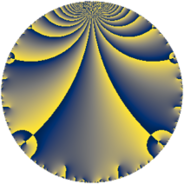# Properties

 Label 294.4.aLevel $294$ Weight $4$ Character orbit 294.a Rep. character $\chi_{294}(1,\cdot)$ Character field $\Q$ Dimension $21$ Newform subspaces $15$ Sturm bound $224$ Trace bound $11$

# Related objects

## Defining parameters

 Level: $$N$$ $$=$$ $$294 = 2 \cdot 3 \cdot 7^{2}$$ Weight: $$k$$ $$=$$ $$4$$ Character orbit: $$[\chi]$$ $$=$$ 294.a (trivial) Character field: $$\Q$$ Newform subspaces: $$15$$ Sturm bound: $$224$$ Trace bound: $$11$$ Distinguishing $$T_p$$: $$5$$, $$11$$

## Dimensions

The following table gives the dimensions of various subspaces of $$M_{4}(\Gamma_0(294))$$.

Total New Old
Modular forms 184 21 163
Cusp forms 152 21 131
Eisenstein series 32 0 32

The following table gives the dimensions of the cuspidal new subspaces with specified eigenvalues for the Atkin-Lehner operators and the Fricke involution.

$$2$$$$3$$$$7$$FrickeDim.
$$+$$$$+$$$$+$$$$+$$$$3$$
$$+$$$$+$$$$-$$$$-$$$$2$$
$$+$$$$-$$$$+$$$$-$$$$3$$
$$+$$$$-$$$$-$$$$+$$$$3$$
$$-$$$$+$$$$+$$$$-$$$$2$$
$$-$$$$+$$$$-$$$$+$$$$3$$
$$-$$$$-$$$$+$$$$+$$$$4$$
$$-$$$$-$$$$-$$$$-$$$$1$$
Plus space$$+$$$$13$$
Minus space$$-$$$$8$$

## Trace form

 $$21q - 2q^{2} + 3q^{3} + 84q^{4} - 26q^{5} - 6q^{6} - 8q^{8} + 189q^{9} + O(q^{10})$$ $$21q - 2q^{2} + 3q^{3} + 84q^{4} - 26q^{5} - 6q^{6} - 8q^{8} + 189q^{9} - 28q^{10} + 68q^{11} + 12q^{12} + 38q^{13} + 66q^{15} + 336q^{16} + 122q^{17} - 18q^{18} + 12q^{19} - 104q^{20} + 112q^{22} - 240q^{23} - 24q^{24} + 447q^{25} + 228q^{26} + 27q^{27} - 202q^{29} + 84q^{30} + 104q^{31} - 32q^{32} - 156q^{33} - 260q^{34} + 756q^{36} + 498q^{37} + 104q^{38} + 978q^{39} - 112q^{40} - 510q^{41} + 328q^{43} + 272q^{44} - 234q^{45} + 640q^{46} + 192q^{47} + 48q^{48} + 1506q^{50} - 414q^{51} + 152q^{52} + 1334q^{53} - 54q^{54} + 1240q^{55} + 384q^{57} + 684q^{58} + 1924q^{59} + 264q^{60} + 758q^{61} - 144q^{62} + 1344q^{64} + 316q^{65} - 456q^{66} - 2304q^{67} + 488q^{68} - 264q^{69} - 1864q^{71} - 72q^{72} - 1646q^{73} - 1724q^{74} + 693q^{75} + 48q^{76} + 84q^{78} - 1784q^{79} - 416q^{80} + 1701q^{81} - 852q^{82} - 772q^{83} - 1412q^{85} - 1032q^{86} - 1014q^{87} + 448q^{88} - 1230q^{89} - 252q^{90} - 960q^{92} - 3804q^{93} - 152q^{95} - 96q^{96} + 106q^{97} + 612q^{99} + O(q^{100})$$

## Decomposition of $$S_{4}^{\mathrm{new}}(\Gamma_0(294))$$ into newform subspaces

Label Dim. $$A$$ Field CM Traces A-L signs $q$-expansion
$$a_2$$ $$a_3$$ $$a_5$$ $$a_7$$ 2 3 7
294.4.a.a $$1$$ $$17.347$$ $$\Q$$ None $$-2$$ $$-3$$ $$-15$$ $$0$$ $$+$$ $$+$$ $$+$$ $$q-2q^{2}-3q^{3}+4q^{4}-15q^{5}+6q^{6}+\cdots$$
294.4.a.b $$1$$ $$17.347$$ $$\Q$$ None $$-2$$ $$-3$$ $$-8$$ $$0$$ $$+$$ $$+$$ $$-$$ $$q-2q^{2}-3q^{3}+4q^{4}-8q^{5}+6q^{6}+\cdots$$
294.4.a.c $$1$$ $$17.347$$ $$\Q$$ None $$-2$$ $$-3$$ $$6$$ $$0$$ $$+$$ $$+$$ $$-$$ $$q-2q^{2}-3q^{3}+4q^{4}+6q^{5}+6q^{6}+\cdots$$
294.4.a.d $$1$$ $$17.347$$ $$\Q$$ None $$-2$$ $$3$$ $$-6$$ $$0$$ $$+$$ $$-$$ $$+$$ $$q-2q^{2}+3q^{3}+4q^{4}-6q^{5}-6q^{6}+\cdots$$
294.4.a.e $$1$$ $$17.347$$ $$\Q$$ None $$-2$$ $$3$$ $$-6$$ $$0$$ $$+$$ $$-$$ $$-$$ $$q-2q^{2}+3q^{3}+4q^{4}-6q^{5}-6q^{6}+\cdots$$
294.4.a.f $$1$$ $$17.347$$ $$\Q$$ None $$-2$$ $$3$$ $$8$$ $$0$$ $$+$$ $$-$$ $$-$$ $$q-2q^{2}+3q^{3}+4q^{4}+8q^{5}-6q^{6}+\cdots$$
294.4.a.g $$1$$ $$17.347$$ $$\Q$$ None $$-2$$ $$3$$ $$15$$ $$0$$ $$+$$ $$-$$ $$-$$ $$q-2q^{2}+3q^{3}+4q^{4}+15q^{5}-6q^{6}+\cdots$$
294.4.a.h $$1$$ $$17.347$$ $$\Q$$ None $$2$$ $$-3$$ $$-2$$ $$0$$ $$-$$ $$+$$ $$-$$ $$q+2q^{2}-3q^{3}+4q^{4}-2q^{5}-6q^{6}+\cdots$$
294.4.a.i $$1$$ $$17.347$$ $$\Q$$ None $$2$$ $$3$$ $$-18$$ $$0$$ $$-$$ $$-$$ $$-$$ $$q+2q^{2}+3q^{3}+4q^{4}-18q^{5}+6q^{6}+\cdots$$
294.4.a.j $$2$$ $$17.347$$ $$\Q(\sqrt{2})$$ None $$-4$$ $$-6$$ $$12$$ $$0$$ $$+$$ $$+$$ $$+$$ $$q-2q^{2}-3q^{3}+4q^{4}+(6+\beta )q^{5}+6q^{6}+\cdots$$
294.4.a.k $$2$$ $$17.347$$ $$\Q(\sqrt{2})$$ None $$-4$$ $$6$$ $$-12$$ $$0$$ $$+$$ $$-$$ $$+$$ $$q-2q^{2}+3q^{3}+4q^{4}+(-6+\beta )q^{5}+\cdots$$
294.4.a.l $$2$$ $$17.347$$ $$\Q(\sqrt{2})$$ None $$4$$ $$-6$$ $$-12$$ $$0$$ $$-$$ $$+$$ $$+$$ $$q+2q^{2}-3q^{3}+4q^{4}+(-6+\beta )q^{5}+\cdots$$
294.4.a.m $$2$$ $$17.347$$ $$\Q(\sqrt{1345})$$ None $$4$$ $$-6$$ $$-5$$ $$0$$ $$-$$ $$+$$ $$-$$ $$q+2q^{2}-3q^{3}+4q^{4}+(-2-\beta )q^{5}+\cdots$$
294.4.a.n $$2$$ $$17.347$$ $$\Q(\sqrt{1345})$$ None $$4$$ $$6$$ $$5$$ $$0$$ $$-$$ $$-$$ $$+$$ $$q+2q^{2}+3q^{3}+4q^{4}+(3-\beta )q^{5}+6q^{6}+\cdots$$
294.4.a.o $$2$$ $$17.347$$ $$\Q(\sqrt{2})$$ None $$4$$ $$6$$ $$12$$ $$0$$ $$-$$ $$-$$ $$+$$ $$q+2q^{2}+3q^{3}+4q^{4}+(6+\beta )q^{5}+6q^{6}+\cdots$$

## Decomposition of $$S_{4}^{\mathrm{old}}(\Gamma_0(294))$$ into lower level spaces

$$S_{4}^{\mathrm{old}}(\Gamma_0(294)) \cong$$ $$S_{4}^{\mathrm{new}}(\Gamma_0(6))$$$$^{\oplus 3}$$$$\oplus$$$$S_{4}^{\mathrm{new}}(\Gamma_0(7))$$$$^{\oplus 8}$$$$\oplus$$$$S_{4}^{\mathrm{new}}(\Gamma_0(14))$$$$^{\oplus 4}$$$$\oplus$$$$S_{4}^{\mathrm{new}}(\Gamma_0(21))$$$$^{\oplus 4}$$$$\oplus$$$$S_{4}^{\mathrm{new}}(\Gamma_0(42))$$$$^{\oplus 2}$$$$\oplus$$$$S_{4}^{\mathrm{new}}(\Gamma_0(49))$$$$^{\oplus 4}$$$$\oplus$$$$S_{4}^{\mathrm{new}}(\Gamma_0(98))$$$$^{\oplus 2}$$$$\oplus$$$$S_{4}^{\mathrm{new}}(\Gamma_0(147))$$$$^{\oplus 2}$$